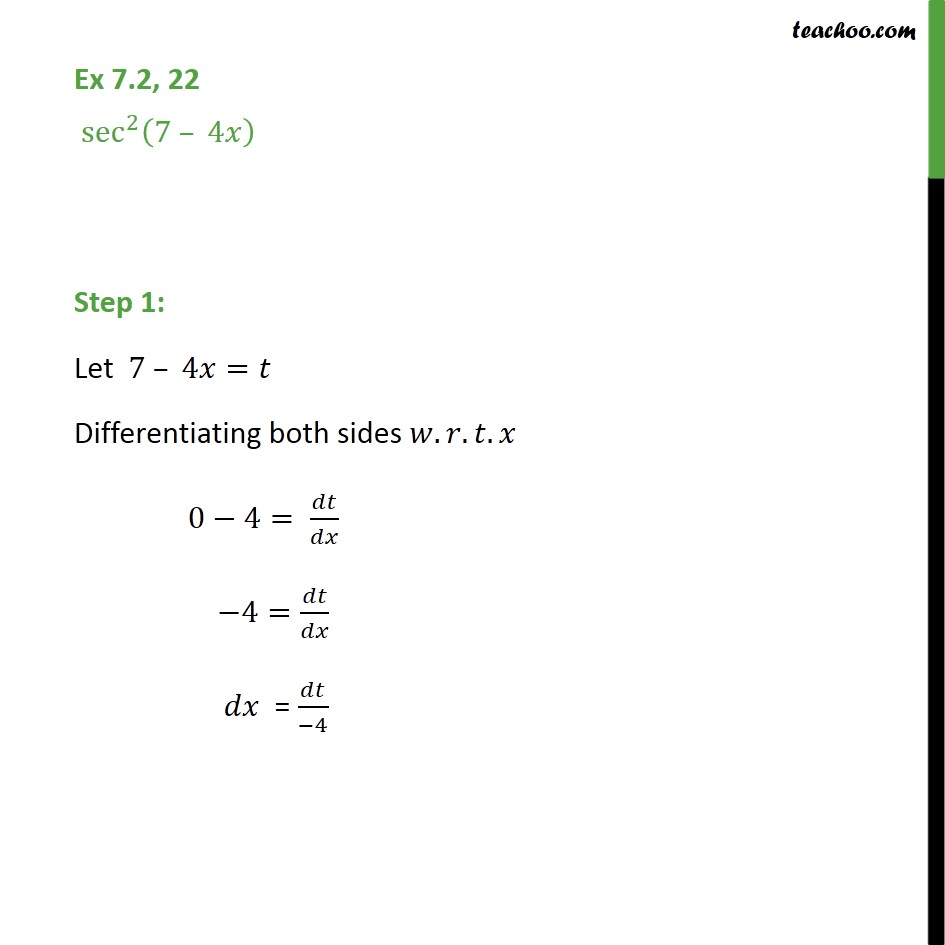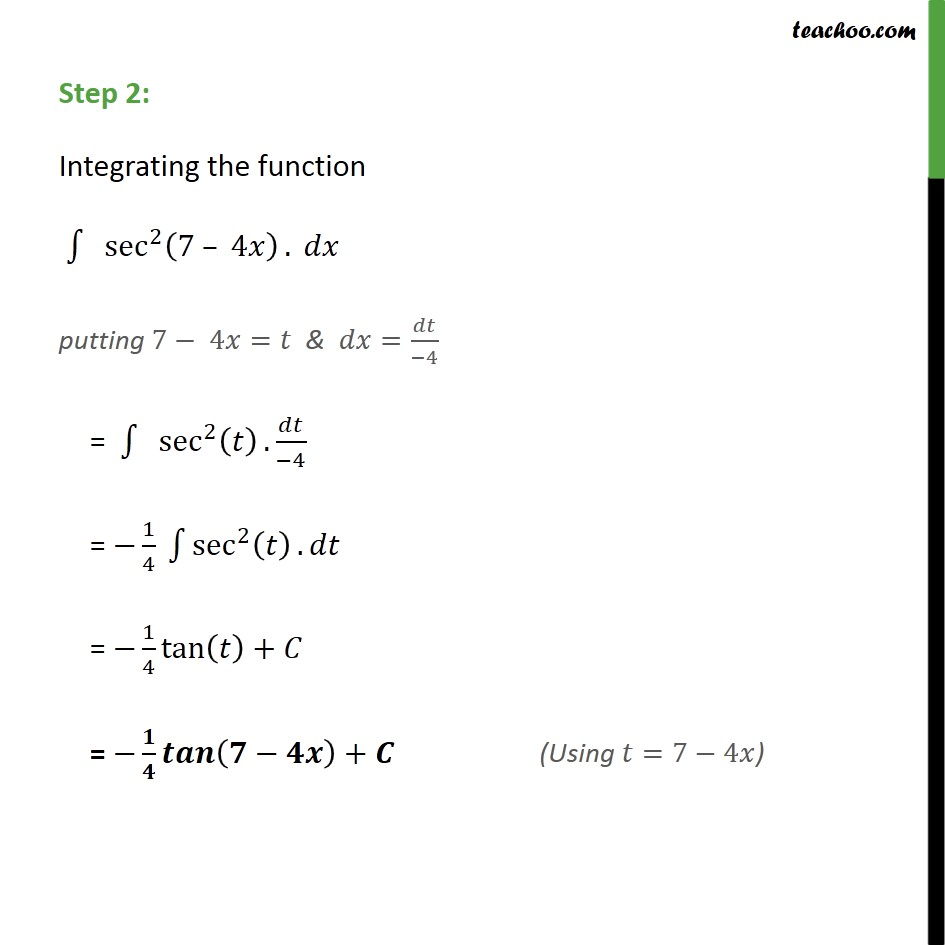1. Chapter 7 Class 12 Integrals (Term 2)
2. Concept wise
3. Integration by substitution - Trignometric - Normal

Transcript

Ex 7.2, 22 sec 2 7 4 Step 1: Let 7 4 = Differentiating both sides . . . 0 4= 4= = 4 Step 2: Integrating the function sec 2 7 4 . putting 7 4 = & = 4 = sec 2 . 4 = 1 4 sec 2 . = 1 4 tan + = +